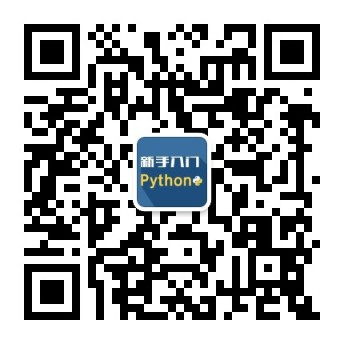2023
03-30

# 编程入门教程Python代码(python编程基础代码)# 计算三角形面积

base = float(input("请输入底边长："))

height = float(input("请输入高："))

area = 0.5 * base * height

print("三角形的面积为：", area)

# 猜数字游戏

import random

number = random.randint(1, 100)

guess = int(input("请猜一个1~100之间的整数："))

while guess != number:

if guess < number:

print("猜小了！")

else:

print("猜大了！")

guess = int(input("请再猜一次："))

print("恭喜你猜对了！")

# 统计单词频率

text = "this is a test text. this is a another test text."

words = text.split()

freq = {}

for word in words:

if word in freq:

freq[word] += 1

else:

freq[word] = 1

print(freq)